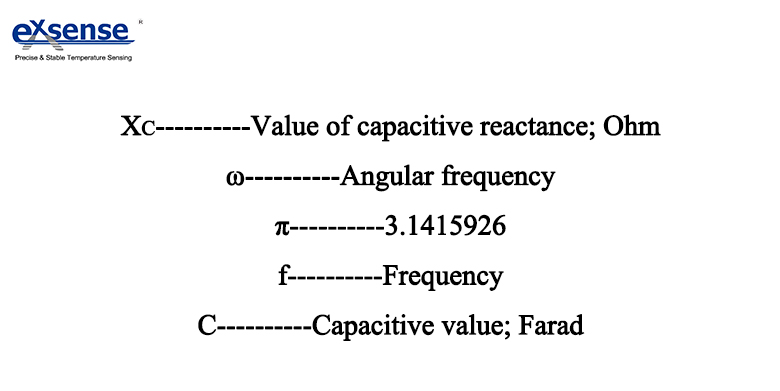QQCustomer Service
Product Catalogue
Tel :
+86-758-2661333
+86-15218448949
Fax :
+86-758-2698912
QQ :
1919442378
No. 201, Shunjing Road, The First Community, Guicheng Street, Dinghu District, Zhaoqing, China, 526070
Technical Support
Frequency Characteristics of Single Layer Capacitor
2019/03/04 03:03:51Chip capacitor--single layer capacitor has the advantages of small size, thin thickness, low equivalent series resistance, lower loss, etc., its frequency characteristic is great, the applying frequency can reach several GHZ, which is suitable for small, microwave occasions, can be used in microwave integrated circuits, play the role of DC isolation, RF bypass, smoothing, tuning and so on.

The frequency characteristic of the capacitor refers to the relationship of the capacitance and other parameters of the capacitor vary with the frequency, so when the capacitor works at high frequency, with the increase of work efficiency, the capacitance will decrease due to the decrease of dielectric coefficient of the insulating medium, and the loss will increase, which will also affect the distribution parameters of the capacitor, and will gradually show sensibility. The frequency characteristic of the capacitor, let's say the angular frequency is ω, the electrostatic capacity of the capacitor is C, then under the ideal condition, the impedance Z of the capacitor (1) can be shown as Formula ①:(1) Ideal Capacitor

①XC=1/(ω*C) =1/(2*π*f*C)As can be seen from the formula ①, the impedance level |Z|, as shown in (2), which tends to decrease in inverse frequency. Due to there is no loss in the ideal capacitor, the equivalent series resistance (ESR) is zero.(2)

But in the actual single layer capacitor (2), in addition to the capacity component C, there is also a resistance (ESR) due to dielectric or electrode loss and a parasitic inductance (ESL) generated by electrodes or wires. As a result, the frequency characteristics of the |Z|, as shown in (4), are V-shaped (some capacitors may become U-shaped) curves, and ESR also shows the frequency characteristics corresponding to the loss value.(3)

The reasons why |Z| and ESR become the curve in (4) are as follows: |Z| of low frequency range is the same as the ideal capacitor, which tends to decrease in inverse frequency. ESR value also shows the characteristic corresponding to the dielectric loss caused by the polarization delay of dielectric medium. Near the point of resonance: the frequency increases, then the |Z| will be affected by ESR from the parasitic inductance or the specific resistance of the electrode,etc., deviating from the ideal capacitor (red dotted line), showing the minimum value. The frequency at which the |Z| is the minimum value is called the self-vibration frequency, at which point |Z|=ESR. If greater than the self-vibration frequency, the component characteristic is converted from capacitors to inductance, |Z| added instead. The range below the self-vibration frequency is called the capacitive field, the opposite is called the perceptual field.(4)

In addition to the influence of dielectric loss, ESR is also affected by the loss of self resistance stroke of the electrode. High Frequency range: In the higher frequency range above the resonance point, the characteristic of the |Z| are determined by the parasitic inductance (L). High Frequency range of The |Z| can be worked out approximately by formula in (2). The trend increases in proportion to the frequency. ESR gradually showed the effect of electrode skin effect and proximity effect.

The above is the frequency characteristics of the actual capacitor. Importantly, the higher the frequency, the less likely it is to ignore the effects of the parasitic component ESR or ESL. As capacitors are increasingly used in high-frequency applications, ESR and ESL are the same as electrostatic capacity, which have become an important parameter for single layer capacitor performance.

Prev : No More

Next : Ceramic Layer Capacitor

mqu.cn site.nuo.cn